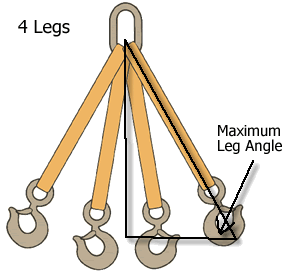# Rated Capacities for Slings

Use the following calculator to determine the rated capacity required by the sling(s).  You will need a sling or slings with a rated capacity equal to or greater than the rated capacity required for the corresponding rigging technique.

Minimum weight on each leg: 0 lbs.
Maximum weight on each leg: 0 lbs.
Minimum Rated Capacity Requred: 0 lbs.

## To calculate the load on a particular sling leg proceed as follows:

1. Take the total weight of the load and divide this by the number of legs supporting the load. For example, assume a 2,000 pound load is to be supported by 2 legs of a sling. The above will give a total minimum weight on each leg of the sling of 2,000 pounds divided by 2 legs or 1,000 pounds.
2. Determine the load factor by observing the load factor chart below. Ex. Assume that the leg angle will be 60°. The load factor for 60° from the load factor chart is 1.154.
3. Multiply the load factor times the minimum weight to be supported by the leg, i.e. 1,000 pounds times 1.154=1154 pounds. The rated sling capacity needed is then 2,308 (1,154x2) pounds in basket capacity to safely lift the 2,000 pound load.